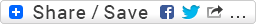## Scheme and Lisp

If you’re coming from Lisp, and then start using Scheme (or the other way around) there are a few small differences between the two languages that it’s useful to always keep in mind. I tried to do that a number of times, but inevitably I find myself once again wondering: how do you say ‘progn’ in scheme?

So, since I recently found online a succinct table that sums up the differences, I thought I’d pass it on to posterity here too.By the way, on the same site the authors report of a small scheme library (initdr.scm) that implements a few very common Lisp functions. Including [dotimes dolist intersection, union set-difference copy-list subset every some copy-tree subst sublis nconc nreverse]… Quite useful too!

### UPDATE 28/11/10:

I realized that most of these lisp functions are already available in Impromptu, under the cl: namespace (check out the common-lisp library functions section on the wiki). Below are just a couple of additions to that, based on the initdr.scm library I was mentioning.. apologies for the confusion!

```;;;;;;;;;;;;;;;;
;; the rest of this is in the cl: impromptu library
;;;;;;;;;;;;;;;;

(define cl:first car)
(define cl:rest cdr)
(define cl:count length)

;; reverse of cons: (cons 'b '(a))
(define cl:l-put
(lambda (obj lst)
(reverse (cons obj (reverse lst)))))

;; dont know why but I like it reversed..
(define cl:nth (lambda (x lst)
(list-ref lst x)))

(define (1+ n) (+ n 1))
(define (1- n) (- n 1))

;; (subst 9 7 '(5 (5 6 7(6 7))))    =>  (5 (5 6 9 (6 9)))
(define (cl:subst new old tree)
(if (pair? tree)
(let ((left (subst new old (car tree)))
(right (subst new old (cdr tree))))
(if (and (eq? left (car tree))
(eq? right (cdr tree)))
tree
(cons left right)))
(if (eqv? old tree)
new
tree)))

;; (sublis '((6 . 9) (7 . 10)) '(5 (5 6 7 (6 7)))))  => (5 (5 9 10 (9 10)))
(define (cl:sublis alist tree)
(if (pair? tree)
(let ((left (sublis alist (car tree)))
(right (sublis alist (cdr tree))))
(if (and (eq? left (car tree))
(eq? right (cdr tree)))
tree
(cons left right)))
(let ((new (assv tree alist)))
(if new
(cdr new)
tree) ) ) )

;; (copy-tree '(5 (5 6 7(6 7))))
(define (cl:copy-tree x)
(if (pair? x)
(cons (copy-tree (car x))
(copy-tree (cdr x)))
x))

; Convert a floating-point number to a string of sign and at most 4 characters.
; Rounds the number so that 1.999 will come out as 2.00 , very small as 0.0 .
; numstring is written assuming that num is not too large or too small,
; i.e. num must be printable in 4 digits.
(define (cl:numstring num)
(let* ((numc (abs num)) (sign (if (< num 0) -1 1)) (exponent 0))
(if (< numc 1.0e-6)
"0.0"
(begin
(if (< numc 1.0)
(begin (while (< numc 100)
(set! numc (* numc 10))
(set! exponent (1- exponent)))
(set! numc (* (round numc) (expt 10 exponent))) )
(set! numc (* numc 1.0001)))
(if (< sign 0)
(string-append "-"
(substring (number->string numc) 0
(min 4 (string-length (number->string numc)))))
(substring (number->string numc) 0
(min 4 (string-length (number->string numc))))) ) ) ))

;(list-flatten '(9 9 (9 9 9 ))))  = (9 9 9 9 9)

(define cl:list-flatten
(lambda (l)
(cond ((null? l)
'())
((atom? l)
(list l))
(#t (append (cl:list-flatten  (car l)) (cl:list-flatten  (cdr l)))))))
```